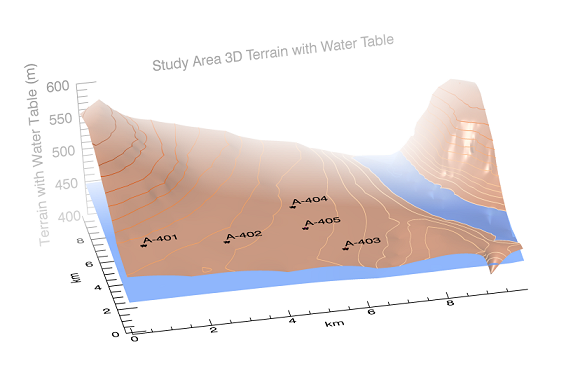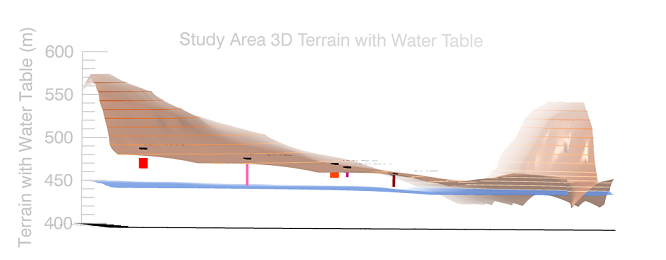In this topic we explore how to combine two different SURFACE plots with POLYLINE and SCATTERPLOT3D to further characterize the study area. We use the same data as in the topic "Model the Study Area and Setting," but view the data as three-dimensional surfaces rather than a two-dimensional contour plot.

Your resulting graphic should look similar to these depending on how you rotate and resize the plot and window (these plots were stretched in the X dimension):## Read In and Grid the Terrain Data

Start by reading in the base data to use throughout the rest of the examples. The data resides in the file TankDataTerrain.csv in the \examples\data directory of your IDL installation. This file contains the surface terrain data of our site, in X, Y, Z coordinates (all in meters). The fourth column of data in this file contains the elevation of the surface of the underlying aquifer.

In this example, we create a template with ASCII_TEMPLATE, then read in the data using READ_ASCII.

`; Create the base template and assign X, Y, Z, `
`; and AQ as the variable names.`
`; Make sure to start the data at row 2 `
`; (row 1 contains column headers).`
`myTemplate = ASCII_TEMPLATE()`
`site = READ_ASCII('TankDataTerrain.csv', \$`
`   TEMPLATE=myTemplate)`

`; Grid the data using the Kriging method `
`; (set DIMENSION to 1000 `
`; to align with the size of the study area). `
`;  Choose another gridding method if you wish.`
`grid = GRIDDATA(site.X, site.Y, site.Z, \$`
`   DIMENSION=1000, METHOD="Kriging")`
` `

## Create the Surface Plot

Next, begin creating the surface plot from the gridded terrain data.

The DEPTH_CUE property creates the haze effect in the graphic, above. We use Gouraud shading in this example, and set ASPECT_Z to 2.5 in order to exaggerate the Z-dimension and make the plot easier to view.

`mySurf = SURFACE(grid, RGB_TABLE=16, TRANSPARENCY=40, \$`
`   COLOR='sienna', DEPTH_CUE=[0,1], SHADING=1, \$`
`   TITLE="Study Area 3D Terrain with Water Table", \$`
`   ASPECT_RATIO=.75, ASPECT_Z=2.5)`
` `
`; Change the direction of the title to be parallel`
`; to the z-azis.`
`mySurf.TITLE.UPDIR = [0,0,1]`
` `
`; Hide the original axes to make viewing the plot easier.`
`mySurf['axis0'].TRANSPARENCY = 100`
`mySurf['axis1'].TRANSPARENCY = 100`
`mySurf['axis2'].TRANSPARENCY = 100`

`; Create alternate axes for easier reading and more accurate `
`; data representation.`
`surfXAxis = AXIS('X', LOCATION=-15, TITLE='km', TICKINTERVAL=2, \$`
`   COORD_TRANSFORM=[0,0.01])`
`surfYAxis = AXIS('Y', LOCATION=-15, TITLE='km', TICKINTERVAL=2, \$`
`   COORD_TRANSFORM=[0,0.01])`
`zAxis = AXIS('Z', LOCATION=[0, 1010], TICKINTERVAL=50, \$`
`   TITLE='Terrain with Water Table (m)', AXIS_RANGE=[0.0,600])`

`; Finally, add the gridded contours leaving off the labels.`
`contours = CONTOUR(grid, C_VALUE=site.Z, PLANAR=0, FONT_SIZE=12, \$`
`   C_LABEL_SHOW=0, /OVERPLOT)`

`; Add the water table: grid the data then plot it with SURFACE.`
`gridH2O = GRIDDATA(site.X, site.Y, site.AQ, DIMENSION=1000, \$`
`   METHOD="Kriging")`
`myWaterTable = SURFACE(gridH2O, TRANSPARENCY=25, \$`
`   COLOR='cornflower', /OVERPLOT)`

## Add the Tank and Well Locations to the Plot

Waste was pumped into both wells and storage tanks, so plot these in 3D space in relation to the surface of the water table. We will also plot the locations of the tanks and wells on the actual SURFACE plot.

`; Plot the tank and well locations in space using POLYLINE.`
`tank1 = POLYLINE([66,66], [210,210], [475,473], /DATA, \$`
`   TARGET=mySurf, COLOR='red', THICK=10)`
`well2 = POLYLINE([276,276], [221,221], [479,450], /DATA, \$`
`   TARGET=mySurf, COLOR='hot pink', THICK=3)`
`well3 = POLYLINE([566,566], [146,146], [463,450], /DATA, \$`
`   TARGET=mySurf, COLOR='dark red', THICK=3)`
`tank4 = POLYLINE([471,471], [483,483], [465,462], /DATA, \$`
`   TARGET=mySurf, COLOR='orange red', THICK=10)`
`well5 = POLYLINE([484,484], [313,313], [470,460], /DATA, \$`
`   TARGET=mySurf, COLOR='medium violet red', THICK=3)`

`; Plot the gridded locations of the wells/tanks, `
`; and labels their location on the SURFACE.`
`xLoc = [66,276,566,471,484]`
`yLoc = [210,221,146,483,313]`
`zLoc = [491,480,463,473,470]`
`zLocLabels = [495,484,467,477,474]`
`labels = ['A-401','A-402','A-403','A-404','A-405']`
` `
`; Use SCATTERPLOT3D to put the locations on the SURFACE`
`; and use TEXT to place the labels.`
`myPlot2 = SCATTERPLOT3D(xLoc, yLoc, zLoc, /OVERPLOT, SYMBOL='*', \$`
`   SYM_SIZE=1, SYM_FILLED=1, SYM_THICK=2, SYM_FILL_COLOR='black')`
`myLabels = TEXT(xLoc, yLoc, zLocLabels, labels, /DATA, /OVERPLOT)`